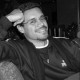You are currently browsing the tag archive for the ‘fermat’ tag.Theorem of the week

I’ve previously written about Fermat’s Little Theorem, which tells us that if $latex p$ is a prime and $latex a$ is not divisible by $latex p$, then $latex a^{p-1} \equiv 1 \pmod{p}$.  That’s not terribly exciting when $latex p = 2$, so let’s concentrate on odd primes $latex p$ in this post.  Then $latex \frac{p-1}{2}$ is a whole number, so it makes sense (in the modular arithmetic world) to let $latex x = a^{\frac{p-1}{2}}$.  Then Fermat‘s Little Theorem tells us that $latex x^2 \equiv 1 \pmod{p}$.

How many possibilities for $latex x$ are there (modulo $latex p$, of course), if we know that $latex x^2 \equiv 1 \pmod{p}$?

Well, that congruence tells us that $latex (x – 1)(x + 1) \equiv 0 \pmod{p}$.  But, in the jargon, “there are no zero-divisors modulo a prime”.  That is, if $latex mn \equiv 0 \pmod{p}$, then $latex m \equiv 0 \pmod{p}$…

View original post 798 more words

Proofs from THE BOOK by Martin Aigner and Günter Ziegler begins by giving six proofs of the infinity of primes. We will go over the second proof. Before we go over this proof, lets cover some background.

— 1. Algebraic Structures —

One of the themes of modern algebra is to compare algebraic structures. An algebraic structure refers to a nonempty set equipped with a binary operation or several binary operations (usually two). Also, we shall refer to a nonempty set equipped with two binary operations satisfying certain properties as a number system. First, we shall define algebraic structures called group, ring and field. A group consists of nonempty set with one binary operation satisfying several conditions. It is the simplest of the three.

Definition 1 Let${G}$ be a nonempty set and${*:G\times G\rightarrow G}$ be a binary operation on${G.}$ Then the set${G}$ together with the binary operation${*}$, denoted${(G,*)}$, is a group, if the following three conditions are satisfied:

i. The binary operation${*}$ is associative, i.e.,${(a*b)*c=a*(b*c)}$ for all${a,b,c\in G}$.

ii. There is a${*}$-identity${e\in G}$, i.e.,${e*x=x*e=x}$ for all${x\in G}$.

iii. For each${a\in G}$, there is a${*}$-inverse${a'\in G}$, i.e.,${a*a'=a'*a=e}$, where${e}$ is the${*}$-identity.

Further more, a group${(G,*)}$ is said to be commutative (or abelian), if the binary operation${*}$ is commutative.

Riker and Picard discuss Fermat’s Last Theorem in “The Royale.” Captain Picard: ‎”For 800 years people have been trying to solve it (Fermat’s Last Theorem)…”

Andrew Wiles did solve it after more than 350 years after Fermat first mentioned the proposition in the margin of his Arithmetica in 1637. Wiles proved FLT in 1994 and it was published in 1995. Apparently, the writers of ST TNG thought this theorem was completely inaccessible.View the video here.

This is a 45 minute documentary [Fermat’s Last Theorem (1996)] about Andrew Wiles, who proved Fermat’s Last Theorem in 1994. The theorem was first conjectured by Pierre de Fermat in 1637 and baffled mathematicians for more than 350 years! It states that no three positive integers$x$,$y$, and$z$ can satisfy the equation$x^n+y^n = z^n$ for$n>2$
The proof relied on elliptic curves and modular forms.